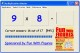Multiplication Master 1.1 Free multiplication tables practice software. For all ages. Freeware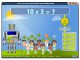Math Games Multiplication 1.1 Practice multiplication (numbers 1 - 10) with the smart robot and his friends. FreewareMaster The Facts Multiplication X 4.4 Master the Facts Multiplication is a no-nonsense drill and practice program to aide students in learning multiplication facts. DemoMultiplication 1.0.4c Multiplication is a simple math multiplication skill for Mac OS X. Freeware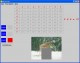Math Genius 1.3.3 The Game lets your child easily learn the Multiplication Table. Shareware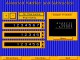Animated Arithmetic 1.0 Teaches addition, subtraction, multiplication and division for 1st - 4th grades. Shareware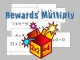Rewards Multiply 2.02 A rewarding game that motivates children to learn their multiplication tables. SharewareAbilities Builder Whole Number Math Facts 6.1 Learn whole number math facts: addition, subtraction, multiplication, division. Demo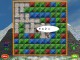Multi Maze Mountain 2 1.1b Multi Maze Mountain is a mazegame dealing with the multiplication tables. SharewareAbilities Builder Math Facts 6.6 Learn whole number math facts addition, subtraction, multiplication, division. Freeware